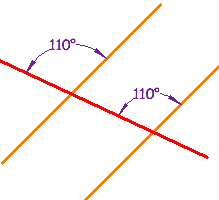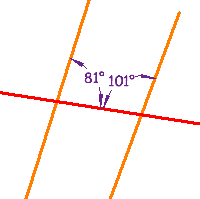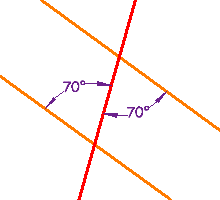# Parallel line

## Pairs of Angles## Testing for Parallel Lines

### Some of those special pairs of angles can be used to test if lines really are parallel:

 If Any Pair Of … Example: Corresponding Angles are equal a = e or Alternate Interior Angles are equal c = f or Alternate Exterior Angles are equal b = g or Consecutive Interior Angles add up to 180° d + f = 180° … then the lines are Parallel## Examples

 These lines are parallel, because a pair ofCorresponding Angles are equal.These lines are not parallel, because a pair ofConsecutive Interior Anglesdo not add up to 180° (81° + 101° =182°) These lines are parallel, because a pair of Alternate Interior Angles are equal